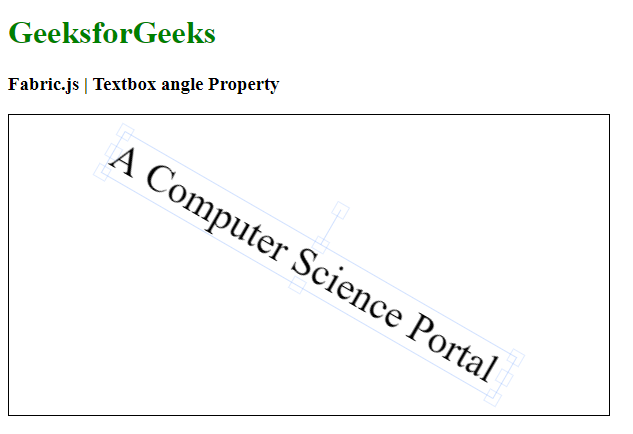# Fabric.js Textbox angle Property

• Last Updated : 20 Jan, 2021

In this article, we are going to see how to set the angle of rotation of an object in the degree of a canvas Textbox using Fabric.js. The Textbox in Fabric.js is movable and can be stretched according to requirement. Further, the rectangle can be customized when it comes to initial stroke color, height, width, fill color, or stroke width.

Approach: To make it possible, we are going to use a JavaScript library called Fabric.js. After importing the library using CDN, we will create a canvas block in the body tag that will contain our Textbox. After this, we will initialize instances of Canvas and Textbox, use the angle property to rotate the text to the required angle and render the Canvas on the Textbox as given in the example below.

Syntax:

```new fabric.Textbox('text', {
angle: number
});```

Parameters: This function accepts a single parameter as mentioned above and described below:

• angle: It specifies the angle of rotation.

Example: This example uses Fabric.js to create a Textbox and make it rotatable.

## HTML

 `<``html``>``<``head``>``    ````    ``<``script` `src``=``"https://cdnjs.cloudflare.com/ajax/libs/fabric.js/4.3.0/fabric.min.js"``>``    ``````<``body``>``    ``<``h1` `style``=``"color: green;"``>``        ``GeeksforGeeks``    ````    ``<``h3``>``        ``Fabric.js | Textbox angle Property``    ````    ``<``canvas` `id``=``"canvas"` `width``=``"600"` `height``=``"300"``        ``style``=``"border:1px solid #000000"``>``    ````    ``<``script``>``        ``// Initiate a Canvas instance ``        ``var canvas = new fabric.Canvas("canvas");`` ` `        ``// Create a new Textbox instance ``        ``var text = new fabric.Textbox(``            ``'A Computer Science Portal', {``            ``width: 450,``            ``angle: 30``        ``});`` ` `        ``// Render the Textbox in canvas ``        ``canvas.add(text);``        ``canvas.centerObject(text);``    ```````

Output:My Personal Notes arrow_drop_up